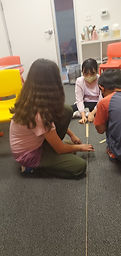Mr. Jake

Target 1​

Lesson Type:

Continuation

Data Probability

:

Interpreting Data

Construct and read graphs from given information to solve problems.

2:

Use a protractor and understanding of percentages to construct pie graphs from given data.

3:

Analyze data presented in pie graphs to answer real-world questions.

7th

Vocabulary:

Pie Chart, Data, Whole

Activities:

Created pie charts based on given information.Home Exploration

Guiding Questions:Absent Students:

Target 2

:

1:

Identify Pi (π) as a constant of all circles.

2:

Explain that Pi (π) is a special number, that continues into infinity without repeating.

3:

Determine that Pi (π) is a ratio of circumference to diameter.

4:

Recognize that Pi (π) is generally represented by 3.14.

5:

Calculate the diameter and circumference of a circle when given the radius (and vice versa).

7th

Vocabulary:

Activities:

Determined the relationship between circumference and diameter.Home Exploration

Guiding Questions:Target 3

:

Vocabulary:

Activities:Home Exploration